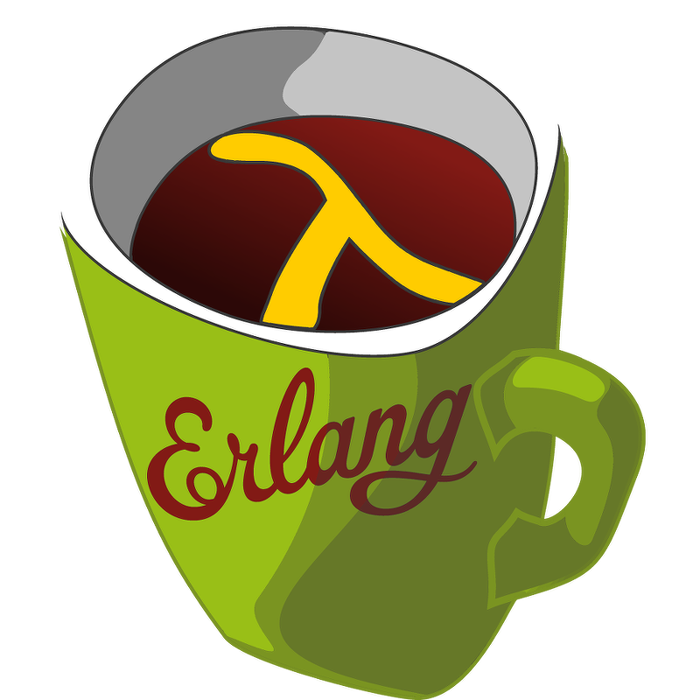This week's LFE Friday was translated with permission from the Erlang Thursday series by Steven Proctor. This week's translator: Robert Virding.

Today's LFE Friday is on digraph:get_cycle/2.

We will continue working with the graph from the previous post on digraph:get_path/3.

``````> (set graph (digraph:new))
#(digraph 8207 12304 16401 true)
> (set v-1 (digraph:add_vertex graph 'v-1))
v-1
> (set v-2 (digraph:add_vertex graph 'v-2))
v-2
> (set v-3 (digraph:add_vertex graph 'v-3))
v-3
> (set v-4 (digraph:add_vertex graph 'v-4))
v-4
> (set e-1 (digraph:add_edge graph v-1 v-2))
(\$e . 0)
> (set e-2 (digraph:add_edge graph v-2 v-3))
(\$e . 1)
> (set e-3 (digraph:add_edge graph v-3 v-4))
(\$e . 2)
> (set e-4 (digraph:add_edge graph v-2 v-4))
(\$e . 3)
> (set e-5 (digraph:add_edge graph v-4 v-1))
(\$e . 4)``````

`digraph:get_cycle/2` takes a graph `G`, and an vertex `V`, and tries to find a path that creates a cycle between the vertex `V` in graph `G`.

``````> (digraph:get_cycle graph v-1)
(v-1 v-2 v-4 v-1)
> (digraph:get_cycle graph v-2)
(v-2 v-4 v-1 v-2)``````

Next, we add a new vertex `v-5`, and a new edge originating from `v-4` and ending on `v-5`

We then call `digraph:get_cycle/2` on `v-5`, and we get back a `false` as no cycle exists in the graph with vertex `v-5` in it.

``````> (set v-5 (digraph:add_vertex graph 'v-5))
v-5
(\$e . 5)
> (digraph:get_cycle graph v-5)
false``````

The `digraph` module also contains the function digraph:get_short_cycle/2.

`digraph:get_short_cycle/2` attempts to find the shortest cycle in the graph `G` for vertex `V`.

The documentation for `digraph:get_short_cycle/2` exact phrasing is:

Tries to find an as short as possible simple cycle through the vertex V of the digraph G.

So depending on how you read that, the shortest cycle might not be guaranteed to be returned, but simply a shorter cycle, which may depend on the overall size and complexity of the graph.

``````> (digraph:get_short_cycle graph v-1)
(v-1 v-2 v-4 v-1)
> (digraph:get_short_cycle graph v-5)
false``````

- Proctor, Robert

16 November 2015

tutorials